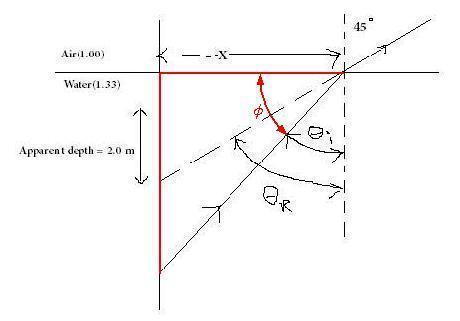Robert is on a ship looking at a school of fish below the lake surface. He estimates the apparent depth of the school to be two meters, and that his observation angle is forty five degrees. Calculate the real depth of the school.
Here is what I think we know:

θr=45°, θi=?, ni=1.00, nr=1.33
Because the index of refraction goes from a lower value to a higher one the light will bend towards the normal which I drew in the diagram.

So if we want to find θi would it be sinθi=((sin45°)(1.33))/(1.00)? I'm a bit lost and would really appreciate some help.

Last edited:

gneill
Mentor
Here is what I think we know:

θr=45°, θi=?, ni=1.00, nr=1.33
Because the index of refraction goes from a lower value to a higher one the light will bend towards the normal which I drew in the diagram.

So if we want to find θi would it be sinθi=((sin45°)(1.33))/(1.00)? I'm a bit lost and would really appreciate some help.

Close. Snell's law says: $\frac{sin(\theta 1)}{sin(\theta 2)} = \frac{n2}{n1}$.

In this case $\theta 1 = 45°~,~n1 = 1.00~,~n2 = 1.33$. It looks like you've got your n1 and n2 swapped.

Then based on that it would be: sinθ2= (1.00/1.33)(sin45) -> θ2=32.1°. Now that I have the angle I can use tanθ=opp/adj correct? But how do I know what the opposite side length is?

gneill
Mentor
Then based on that it would be: sinθ2= (1.00/1.33)(sin45) -> θ2=32.1°. Now that I have the angle I can use tanθ=opp/adj correct? But how do I know what the opposite side length is?

If you know θ2 (which is θi on your diagram) then you can calculate the angle $\phi$ between the horizontal water surface and the light ray. What triangle side length is the same regardless of the depth?#### Attachments

The horizontal side x does not change. Could you find the angle by subtracting θ2 from 90° which would make ϕ 58°?

gneill
Mentor
The horizontal side x does not change. Could you find the angle by subtracting θ2 from 90° which would make ϕ 58°?

That's the idea!

So could I do the same thing to find x by subtracting 45° from 90° which is 45° and if the length of the apparent depth is 2 meters then x must also be 2 because 45-45-90 triangles have legs of equal length?

gneill
Mentor
So could I do the same thing to find x by subtracting 45° from 90° which is 45° and if the length of the apparent depth is 2 meters then x must also be 2 because 45-45-90 triangles have legs of equal length?

Absolutely!

our real depth then is 2/Tan32.1=3.19 m. Thank you so much for your help.